Ex 8.1

Chapter 8 Class 9 Quadrilaterals
Serial order wise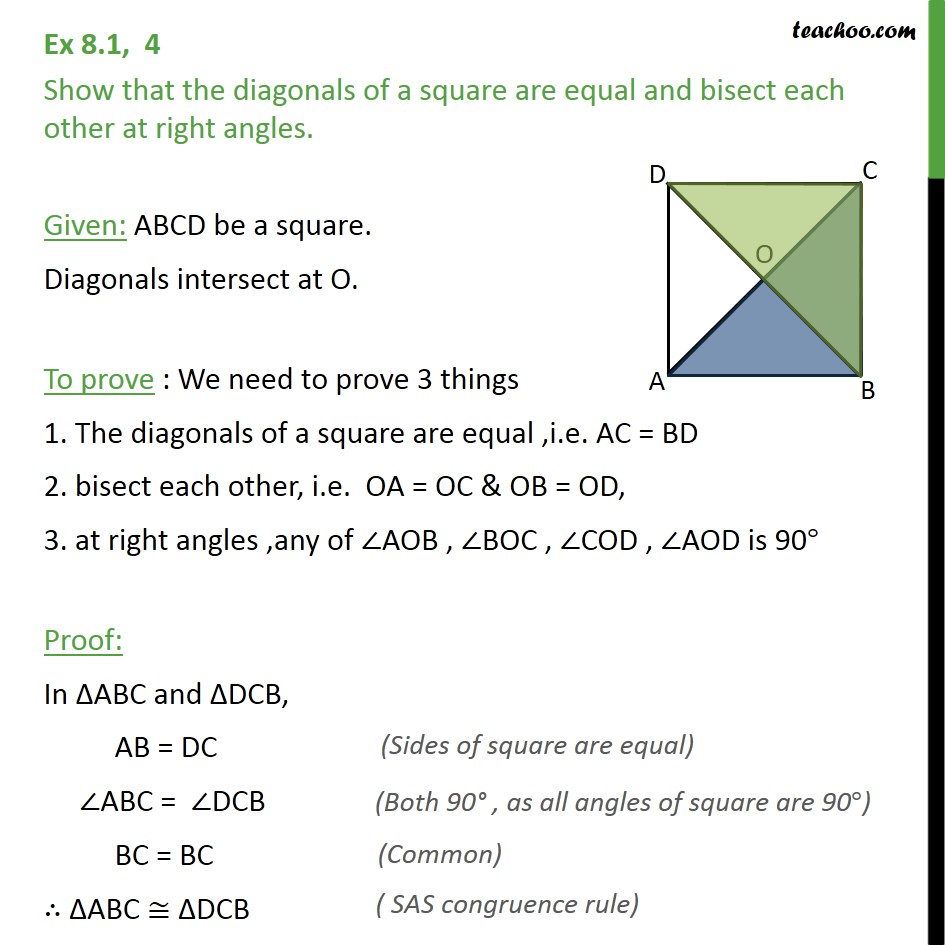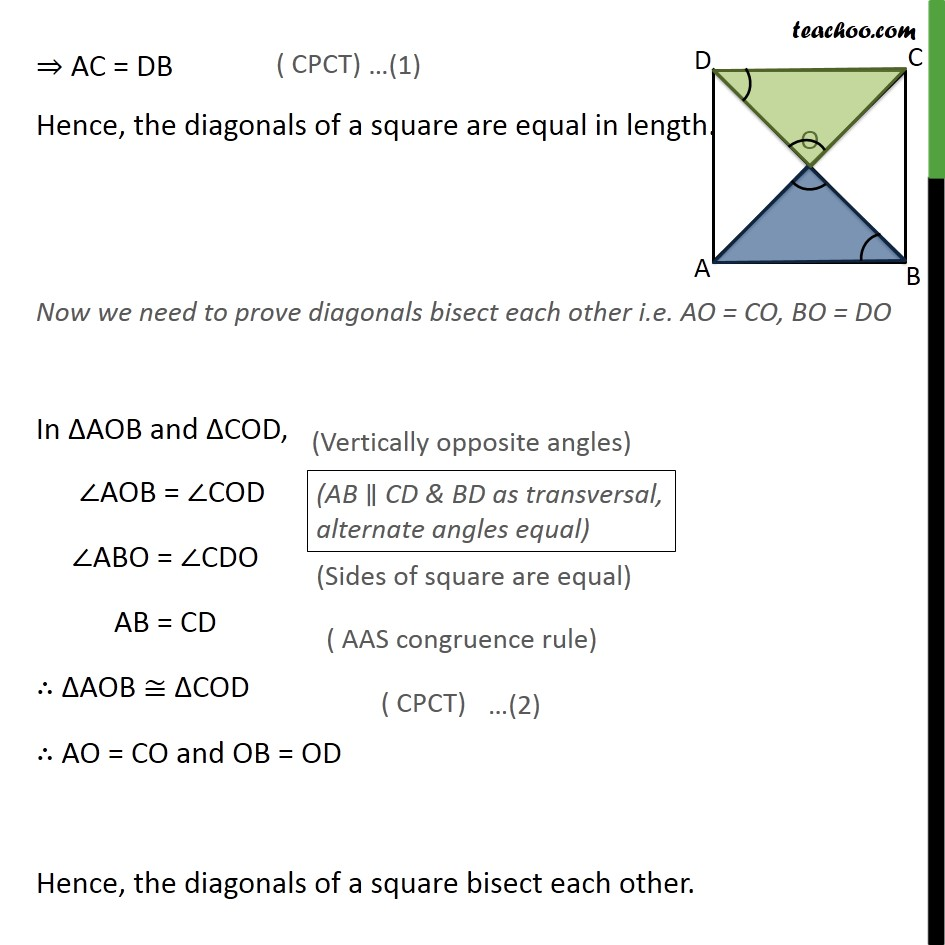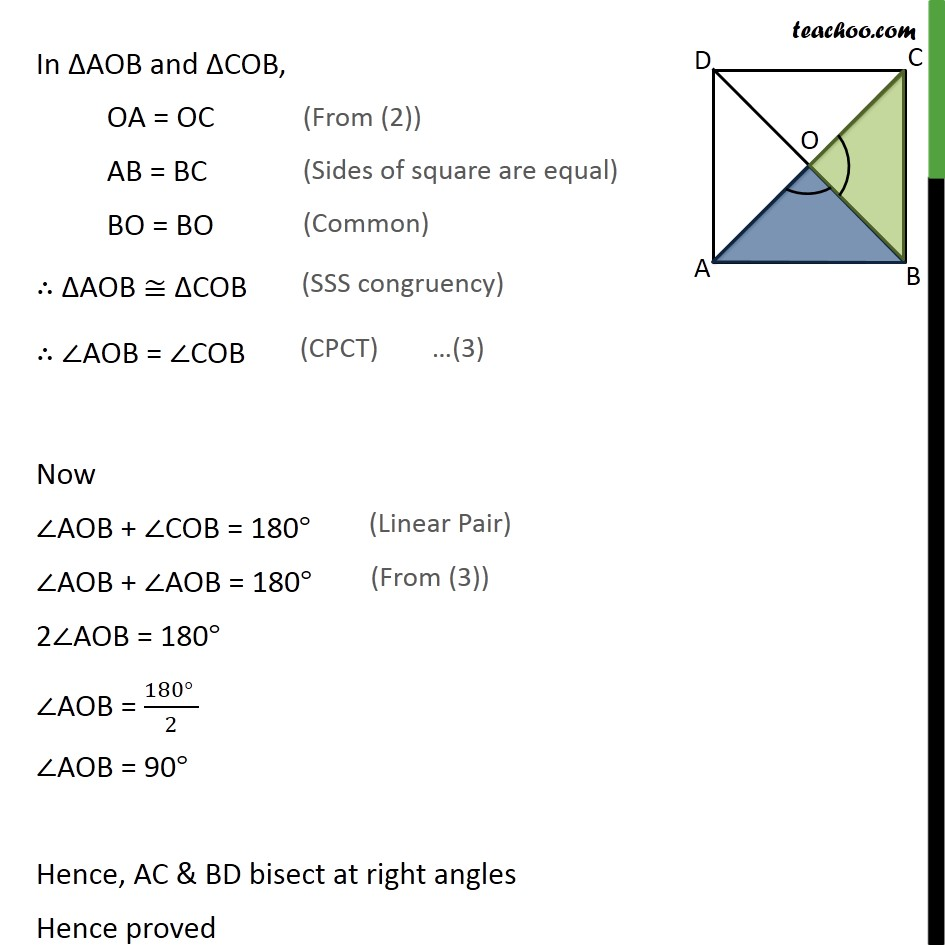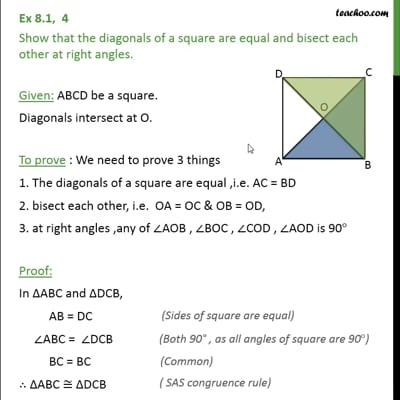This video is only available for Teachoo black users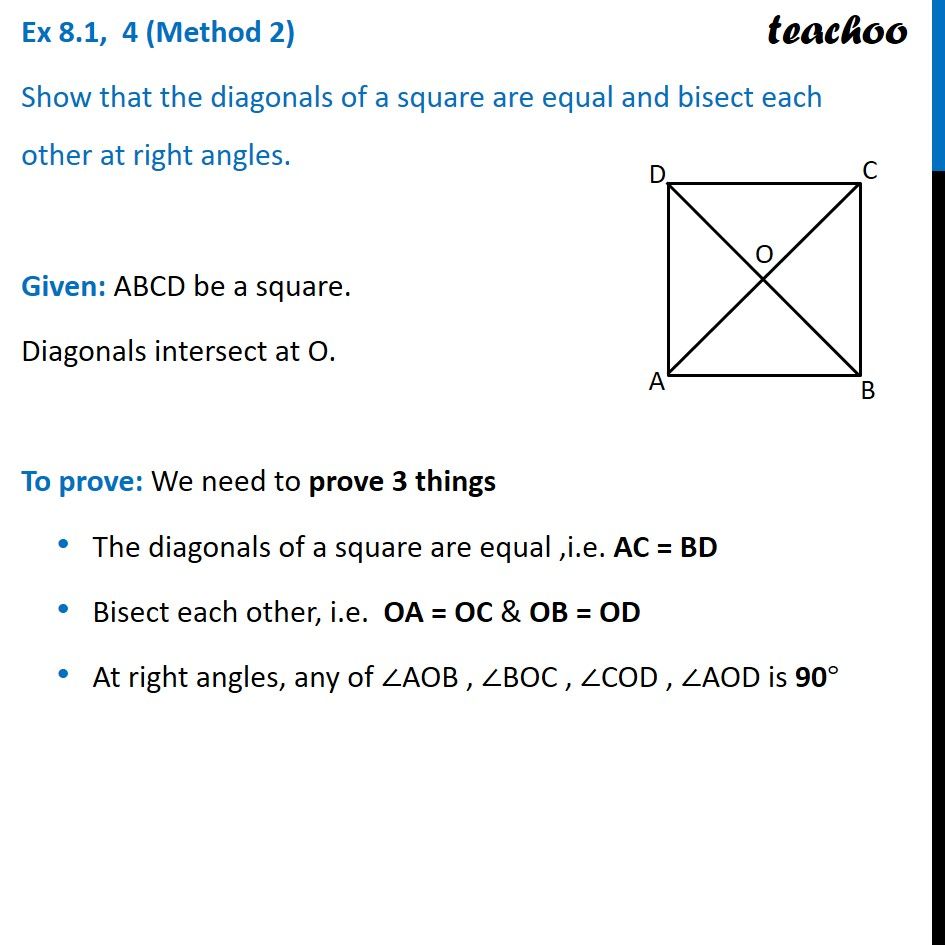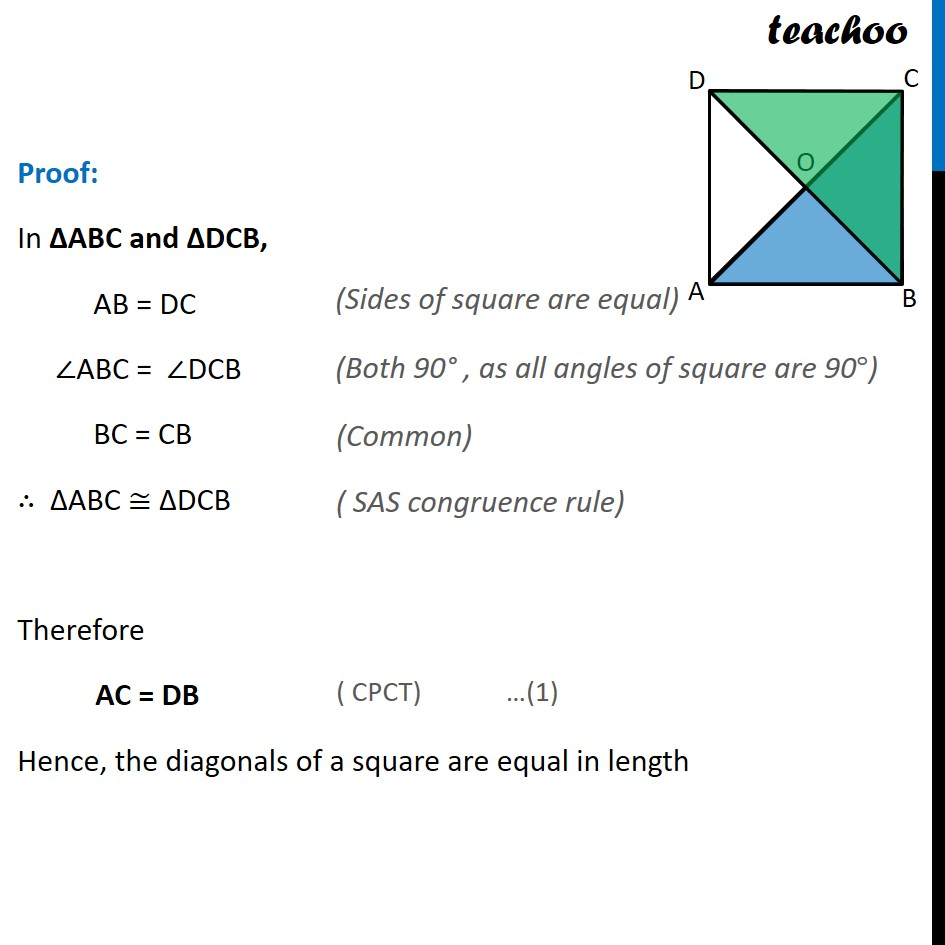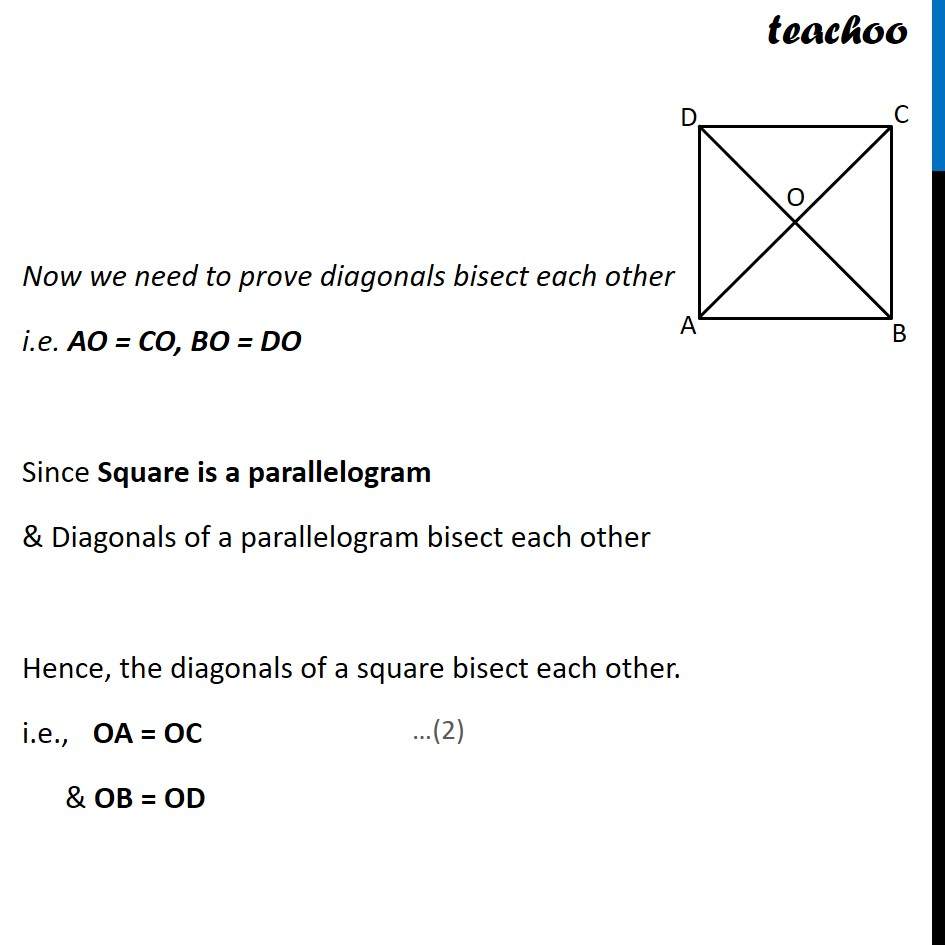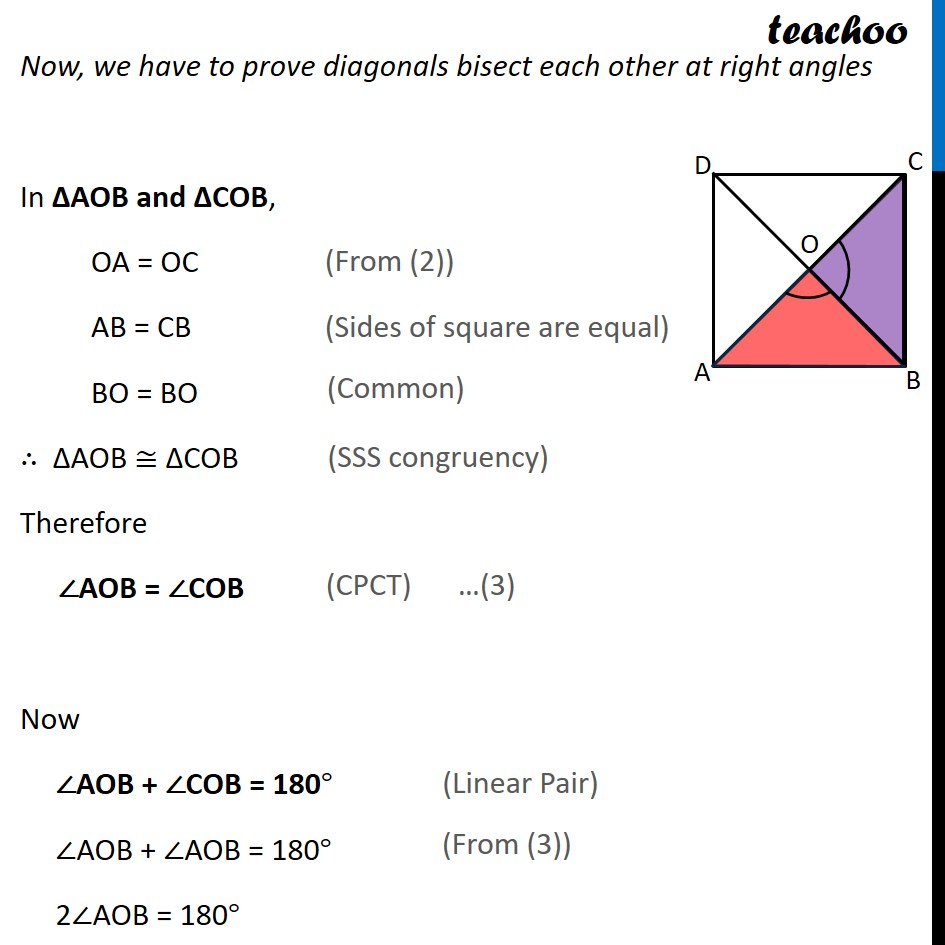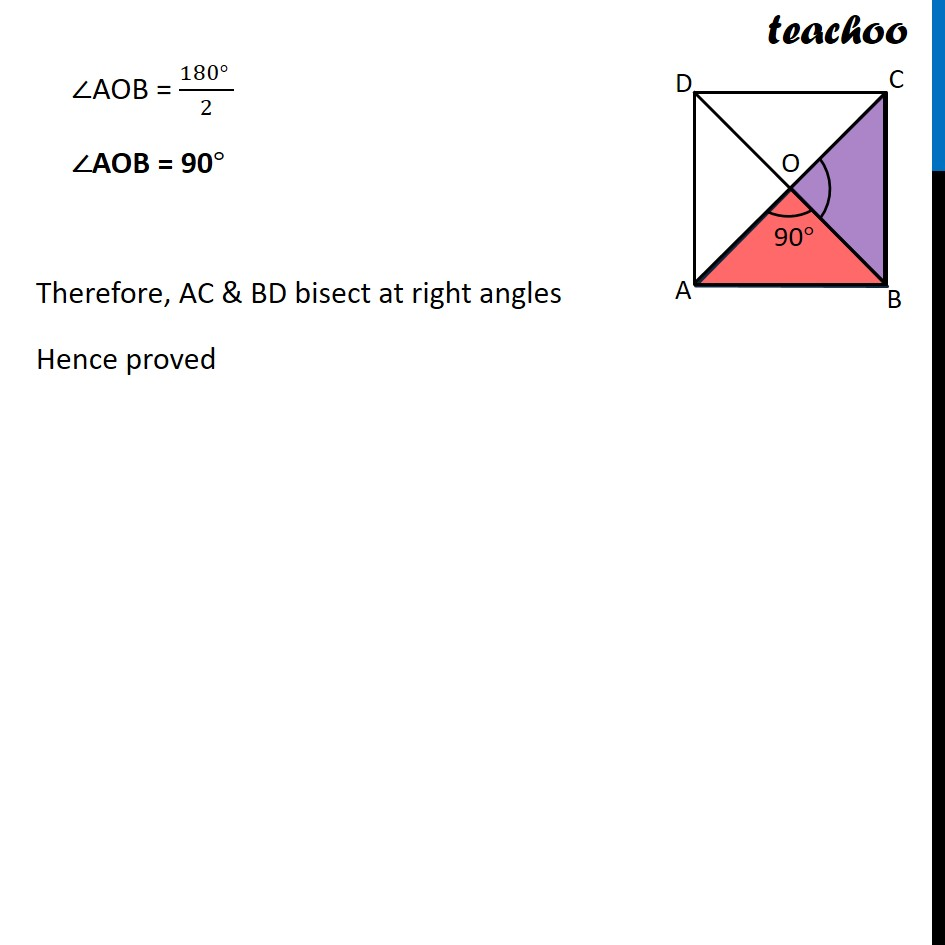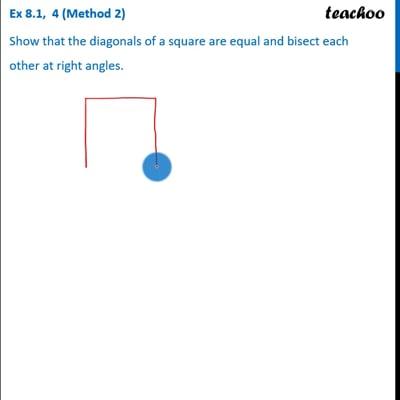This video is only available for Teachoo black users

Solve all your doubts with Teachoo Black (new monthly pack available now!)

### Transcript

Ex 8.1, 4 Show that the diagonals of a square are equal and bisect each other at right angles. Given: ABCD be a square. Diagonals intersect at O. To prove : We need to prove 3 things 1. The diagonals of a square are equal ,i.e. AC = BD 2. bisect each other, i.e. OA = OC & OB = OD, 3. at right angles ,any of AOB , BOC , COD , AOD is 90 Proof: In ABC and DCB, AB = DC ABC = DCB BC = BC ABC DCB AC = DB Hence, the diagonals of a square are equal in length. Now we need to prove diagonals bisect each other i.e. AO = CO, BO = DO In AOB and COD, AOB = COD ABO = CDO AB = CD AOB COD AO = CO and OB = OD Hence, the diagonals of a square bisect each other. In AOB and COB, OA = OC AB = BC BO = BO AOB COB AOB = COB Now AOB + COB = 180 AOB + AOB = 180 2 AOB = 180 AOB = (180 )/2 AOB = 90 Hence, AC & BD bisect at right angles Hence proved Ex 8.1, 4 (Method 2) Show that the diagonals of a square are equal and bisect each other at right angles. Ex 8.1, 4 (Method 2) Show that the diagonals of a square are equal and bisect each other at right angles. Given: ABCD be a square. Diagonals intersect at O. To prove: We need to prove 3 things The diagonals of a square are equal ,i.e. AC = BD Bisect each other, i.e. OA = OC & OB = OD At right angles, any of ∠AOB , ∠BOC , ∠COD , ∠AOD is 90° Proof: In ΔABC and ΔDCB, AB = DC ∠ABC = ∠DCB BC = CB ∴ ΔABC ≅ ΔDCB Therefore AC = DB Hence, the diagonals of a square are equal in length (Sides of square are equal) (Both 90° , as all angles of square are 90°) (Common) ( SAS congruence rule) ( CPCT) …(1) Now we need to prove diagonals bisect each other i.e. AO = CO, BO = DO Since Square is a parallelogram & Diagonals of a parallelogram bisect each other Hence, the diagonals of a square bisect each other. i.e., OA = OC & OB = OD Now, we have to prove diagonals bisect each other at right angles In ΔAOB and ΔCOB, OA = OC AB = CB BO = BO ∴ ΔAOB ≅ ΔCOB Therefore ∠AOB = ∠COB Now ∠AOB + ∠COB = 180° ∠AOB + ∠AOB = 180° 2∠AOB = 180° (From (2)) (Sides of square are equal) (Common) (SSS congruency) (CPCT) (Linear Pair) (From (3)) ∠AOB = (180° )/2 ∠AOB = 90° Therefore, AC & BD bisect at right angles Hence proved